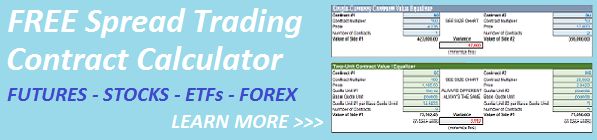Select Page< Back to Intro

### How to Equalize Spread Legs When Both Securities Are in the Same Currency and Have the Same Quote Units

This is the base case where both securities are in the same currency, no currency transformation is required and the Quote Units of the two securities are the same.

Suppose you are spread trading two equity index futures listed on the CME, the full size NASDAQ 100 future (ticker ND) and the full size S&P 500 future (SP).  Both are denominated in USD.

For our example, let’s use these values:

• Currency = USD on both legs
• Currency Value vs. Base Currency (your trading currency) = not required
• Quote Unit = price points on both legs
• Minimum Tick Value = 0.25 ND and 0.1 SP
• Contract Multiplier = 100 ND and 250 SP
• Price = 4236 ND and 2019 SP

Here’s the contract calculation to normalize both legs.  Simply adjust the number of contracts on each leg to minimize the absolute variance in net exposures.

ND leg exposure = 100 x 4236 x contracts

SP leg exposure = 250 x 2019 x contracts

If the two legs are cash securities (stocks, funds, ETFs) rather than futures contracts, always use 1 for the contract multiplier.  If one leg is a future and the other is a cash security, use the contract multiplier for the future and 1 for the cash security.  This only works if the minimum purchase or sale size of your cash security position is individual security units, rather than baskets or bundles of securities, otherwise use the basket or bundle multiplier as the contract multiplier for the cash security leg.

Continue to Case 2:  How to Equalize Contracts with Different Quote Units >